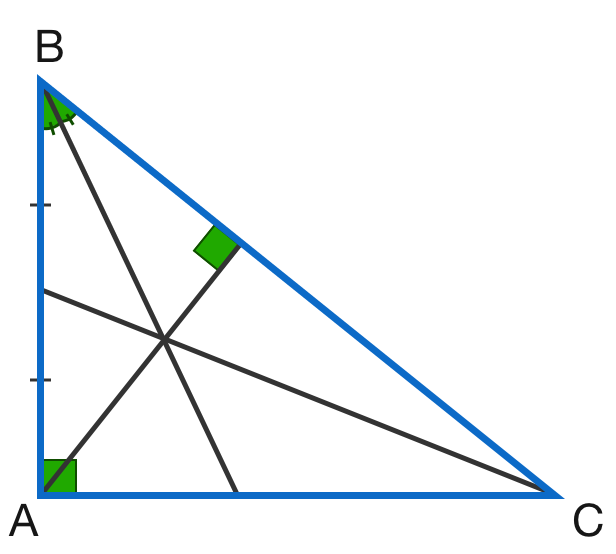# Centroid, Incentre, Orthocentre?

Geometry Level 5As shown above, an angle bisector, median, and altitude intersect at the same point in right triangle $ABC.$

$\cos^2 \angle ABC = \dfrac{p}{q+\sqrt{r}},$ where $p$ and $q$ are positive integers and $r$ is a prime number. Find the value of $p+q+r$.

×

Problem Loading...

Note Loading...

Set Loading...